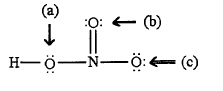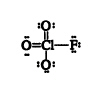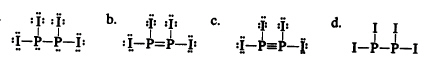Name:    Practice Final A

Multiple Choice
Identify the choice that best completes the statement or answers the question.

1.

Calculate the vapor pressure of a solution prepared by dissolving 0.75 mol of Safrole, a non-volatile solute, in 950 g of ethanol (C2H5OH) at 25 °C.  (P°ethanol = 50.0 torr at 25 °C)
 A) 1.8 torr B) 11 torr C) 40 torr D) 48 torr

2.

Which of the following compounds is polar?
 A) KrF2 B) CO2 C) BeCl2 D) SF2

3.

What is the molecular shape of NH2Cl as predicted by the VSEPR theory?
 A) tetrahedral B) trigonal planar C) T-shaped D) trigonal pyramidal

4.

The bond energy for the diatomic molecule XY is greater than that of VW.  Which of the following statements is accurate?
 A) The XY bond length is shorter than the VW bond. B) The atomic radius of X must be greater than that of V. C) The electronegativity difference between X and Y is greater than tht of V and W. D) The VW bond is more polar than the XY bond.

5.

Which of the following indicates the existence of strong intermolecular forces in a liquid?
 A) a very low boiling point B) a very low viscosity C) a very low vapor pressure D) a very low heat of vaporization

6.

Which of the following correctly lists the compounds in order of increasing boiling point?
 A) CH3CH2OH  <  CH3CH2OCH3  <  CH3CH2CH2OH B) CH3CH2OCH3  <  CH3CH2OH  <  CH3CH2CH2OH C) CH3CH2CH2OH  <  CH3CH2OH  <  CH3CH2OCH3 D) CH3CH2CH2OH  <  CH3CH2OCH3  <  CH3CH2OH

7.

Which of the following best represents the formal charges on the structure shown below:A) +1 for H, –2 for each oxygen, +5 for N B) 0 for all atoms C) +1 for N, –1 for oxygen (c), 0 for all other atoms D) +1 for N and H, –1 for oxygen (a) and (c), 0 for oxygen (b)

8.

Which of the following substances would be expected to have the highest viscosity at room temperature?
 A) C4H10 B) HOCH2CH2OH C) C8H18 D) C2H5NH2

9.

Which of the following would be expected to have the highest boiling point?
 A) CBr4 B) CI4 C) CCl4 D) CF4

10.

What is the molecular shape of NOCl as predicted by the VSEPR theory?
 A) tetrahedral B) trigonal planar C) linear D) bent

11.

If 20.0 g of urea [CO(NH2)2] is dissolved in 25.0 g of water, what is the freezing point of the solution? (Kf = 1.86 °C/m)
 A) –1.40 °C B) –3.72 °C C) –2.47 °C D) –24.7 °C

12.

What is the molecular shape for ClF3 as predicted by the VSEPR model?
 A) T-shaped B) trigonal pyramidal C) trigonal planar D) see-saw

13.

Which of the following compounds displays the most ionic character it its bonds?
 A) CO2 B) H2O C) HF D) NO2

14.

What is the approximate bond angle in AsCl3?
 A) 180 B) 90 C) 120 D) 109

15.

Octane (C8H18) becomes a liquid because of
 A) dipole-dipole forces B) hydrogen bonding C) covalent bonding D) London forces

16.

In the following Lewis structure for ClO3F, chlorine has a formal charge of ______ and an oxidation number of _____.A) 7, –1 B) 1, 1 C) 1, –1 D) 1, 7

17.

Select the correct Lewis structure for P2I4.A) a B) b C) c D) d